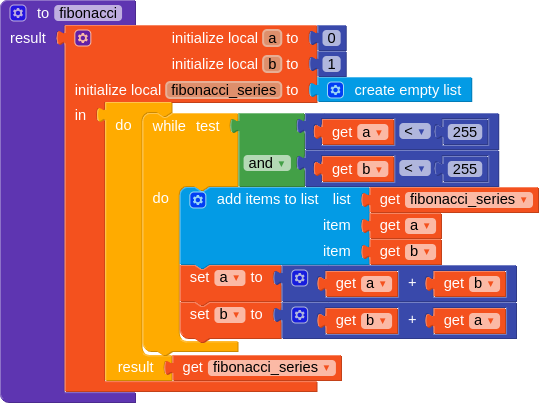# Daily Challenge #14

This is your quest for today:

The Fibonacci Sequence is the sequence of numbers (Fibonacci Numbers) whose sum is the two preceding numbers (e.g. 0, 1, 1, 2, 3, etc). Using 0 and 1 as the starting values, create a procedure that returns an result containing all of the Fibonacci numbers less than 255.

3 Likes
``````def fibonacci():
a = 0
b = 1
while a < 255 and b < 255:
print (a, "\n", b)
a = a + b
b = b + a
fibonacci()
``````
``````Output:

0
1
1
2
3
5
8
13
21
34
55
89
144
233
``````Edit 1: Replaced code with working Python code.
Edit 2: Debugged Python code.
Edit 3: Added equivalent blockly code.

7 Likes

Another approach using recursion:

Output:Blocks:

8 Likes

You’d need to start from 0 and 1, according to Peter’s challenge instructions.3 Likes